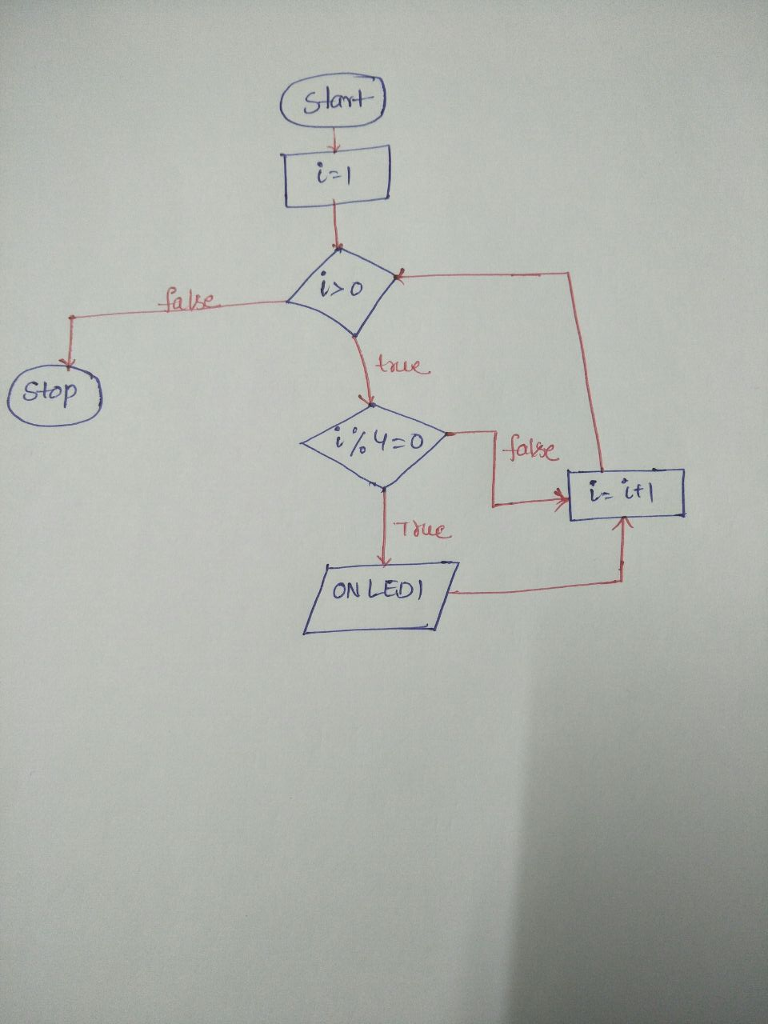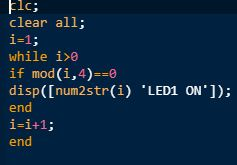# Homework Solution: HW02-1 Develop an algorithm which generates an infinite loop and turns ON (i.e., T…

HW02-1 Develop an algorithm which generates an infinite loop and turns ON (i.e., True, Hi, or Logic 1) a Boolean Variable, LED1, once every fourth iteration. Write a flowchart Matlab script which generates an infinite loop in Matlab.  Document this algorithm using a flowchart or pseudo-code. o For a flowchart, use an online tool such as “draw.io” (or powerpoint). Hand- written drawings (sketches) are permitted for In-Class Activities (for time/expediency) but ARE NOT acceptable for this HW assignment. o For pseudo-code, use a simple text file and standard pseudo-code protocol.  Use the variable names specified and document (explain) any additional variables.  Submit this plan as a separate file (HW02-1.io, or HW02-1.ppt, or HW02-1.txt, etc.). HW02-2 Write a Matlab function which implements the algorithm developed in HW02-1.  Document this function with Matlab comments. This will help you when you implement this algorithm in other programming languages, such as EmbeddedC.  Submit this Matlab function as a separate file (HW02-2.m)  Capture the 5-10 lines of actual output of this function to demonstrate its correct operation. Save this output as a text file or pdf file (converted from an image).  Submit this output as a separate file (HW02-2.txt or HW02-2.pdf).